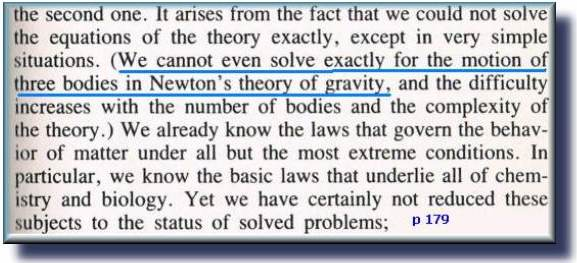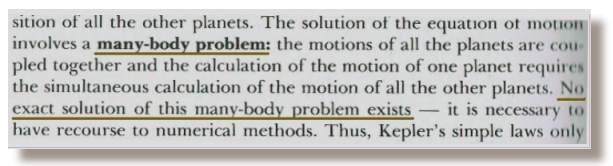Many Body Problem Jonathan Ainsley Bain

 The many-body problem is a computational problem that I had solved in 2008. Long before I realized it was a problem. Solution to the many-body-problem (Simply): Store all the potential momentums for each body in a ‘holding matrix’ before even beginning to add them to each or any of the existing positions.

 If we have 10 bodies under gravity, then there are 90 gravitational calculations. Instead of adding in the new positioning one body at a time; all the new additions to momentums are calculated and then stored in a holding matrix for momentum which has 90 ‘new momentum changes’. Only once all the 90 new movements have been determined (and stored separately) does the software then begin to add those new momentums to the old momentums. Then; only when all those calculations have been completed, does the program begin to calculate the new movements to each of the old positions. After that is complete the timer can start a new quantum of time, and the calculation repeats; one Iota at a time. At least two separate steps are required. I use three steps. Each step is completed before the next one can begin. Do not calculate entire objects one at a time. Calculate all the momentum changes; then calculate all the new momentums; and then calculate all the new positions. The holding matrix method is not only accurate, but it is the only way to construct the algorithm. So it would make zero difference which planet or star was calculated first, because all new calculated momentums are not added to the real position until all the new momentums for that split second of time have been finalized. Is this the same problem that led Hawking to believe there was not enough computing power for even three bodies under Newtonian gravity back in the eighties? I think so. If one can calculate one object, then two … three or four are not much different. The time taken for each calculation does increase to the power 2. Hawking’s problem was simply one of computational process. The many-body solution being that one must philosophically split quantum time. That would explain why a self-taught computer programmer can solve physics problems that trained Physicists can not. The computer just will not do it any other way. It’s quite a boast, eh? Not really. It’s not a boast, just a calculation with a logical conclusion. It cannot be any other way than what it is. To claim anything less would be lying. The computer is an unforgiving, and a perfect mathematics teacher. Or perhaps I was just ignorant enough to know that I was not supposed to be able to answer the question? But I only figured out how the timer works because I had been having great fun making a game of computer cricket. All my colleagues have a fear of timers. They all prefer to make boring business applications. Real time computations have made most of calculus obsolescent. A similar (but trickier) process to the many-body solution is required when joining many masses together and having them rip apart under strong gravity. The sequence must be calculated in such a way that every new event must be pre-calculated before it is actually calculated so that the new changes do not effect the old positioning. Calculations from one instance of time cannot interfere with another instance of time until all the potential movements of all the objects have been calculated and all the results placed in a temporary matrix (sum of potentials). This method holds true beyond just gravity calculations. It would apply to chemistry, electromagnetic calculations, and even biological modeling as well..! There is a complex chasm of mathematics between each iota of quantum time in the universe. This algorithm is such that if A represents all the particles in the universe, then the number of gravitational calculations is equal to (A^2)-A. 100 objects require 9900 calculations per time unit. Every single particle’s interaction with every other particle (gravitational or electromagnetic or otherwise) must be pre-calculated before any particle can begin to be effected. We have to actually split each quanta of time in half, so that all new momentums are calculated (and stored aside) before the first actual motion can be performed. Seeing as though I feel the need for verbosity right now, let me just re-copy the quote from that deeply ironic title: ‘A Brief History of Time’Of course, nothing is exact. However there is the same accuracy with one body or with 100 bodies. It just takes about 9 900 times longer to calculate the interacting gravity fields of 100 bodies. This from the Ohanian textbook on Physics:I shall happily leave this chapter full of questions and a few positivist answers… < discuss the many-body-problem on a forum > Latest Article: < gravitational waves & general relativity > A computational analysis of the theory of gravitational waves; as expressed within Einstein’s Theory of General Relativity and the wider realm of Astrophysics. Essentially a critique, this study has been written for the purpose of explaining the unobvious challenges faced in building graphically dynamic evolutionary computer models. These models compute the theoretical functionality of gravitational waves in the celestial paradigms of solar system formation and galaxy formation. This solution to the Many Body Problem has been used to calculate the answers to: < rubin's problem - the rotation curves of galaxies > < why the moon is receding from the earth > < how the solar system formed > < relativity revised > < quantum gravity > < zeno and planck > < dark energy > < dark matter > < a summary of the force of gravity >

 Click the link below and Download Orbit Game 7    (2014) to see the real-time computations of the many-body problem. Version 7 allows for planet sizes to change via collisions with each other. >> orbit-game-7.exe << If your PC uses the comma as a decimal separator, then these apps won't work so good. .< top > < why? > < a dream >